#2018 iT 邦幫忙鐵人賽DAY 27
4
Modern Web

## [PixiJS - Day-27] 實作：第一版萬花筒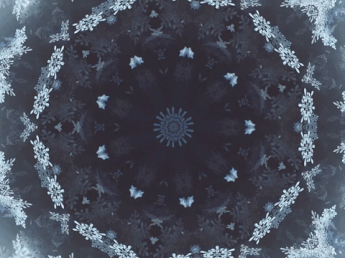Step1. 畫出一片三角形與內容：

Step2. 複製滿一圈

Step3. 做動態

Step1. 畫出一片三角形與內容：``````var triangle = new PIXI.Graphics();
triangle.beginFill(0x000000, 0.3);
triangle.moveTo(0,0);
triangle.lineTo(
Math.cos( 0 * ( 60 ) * (Math.PI / 180)) * 100,
Math.sin( 0 * ( 60 ) * (Math.PI / 180)) * 100
);
triangle.lineTo(
Math.cos( 1 * ( 60 ) * (Math.PI / 180)) * 100,
Math.sin( 1 * ( 60 ) * (Math.PI / 180)) * 100
);
triangle.lineTo(0,0);
````````````var bunny = PIXI.Sprite.fromImage('bunny.png');
``````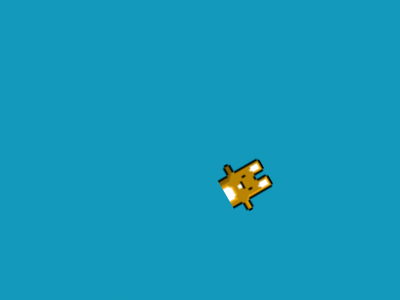``````//將剛剛畫的三角形當做兔子的遮罩
``````

``````// new 一個容器
var container = new PIXI.Container();
// 把容器放進場景
// 把三角形放進容器
// 把兔子放進容器
``````

Step2. 複製滿一圈：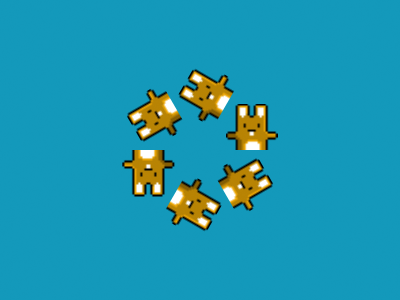``````for(var i = 0; i<6; i++){
var container = new PIXI.Container();
container.rotation = i * ( 60 ) * (Math.PI / 180);
...
}
``````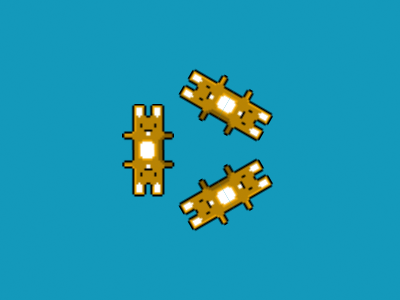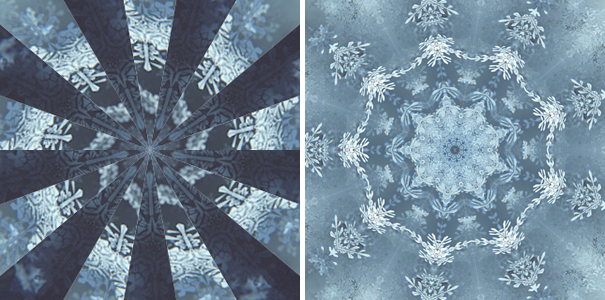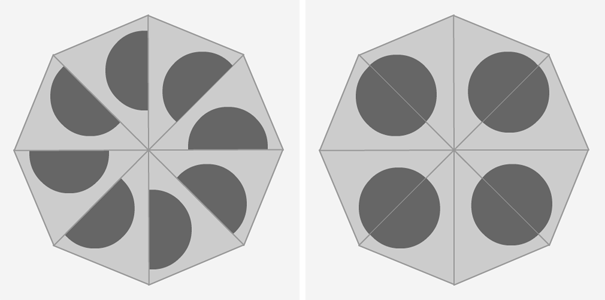Step3. 做動態：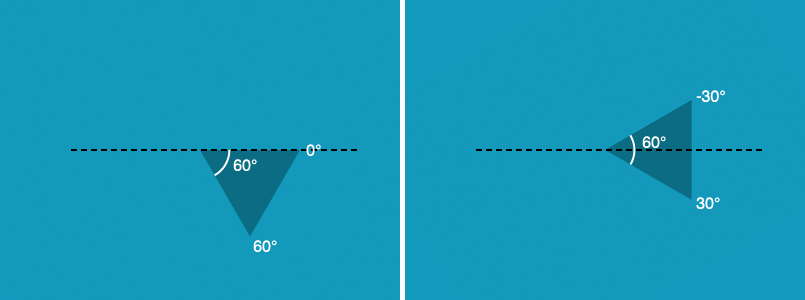0